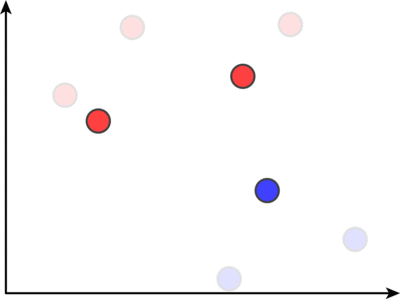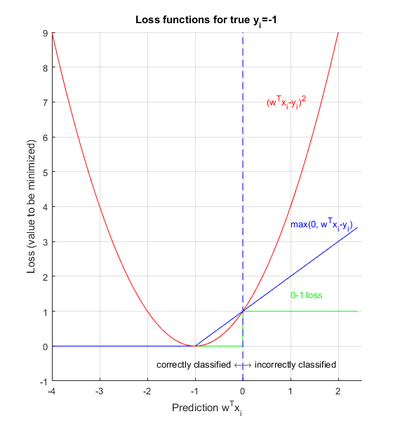# Course:CPSC522/Support Vector Machines

Jump to navigation Jump to search

## Support Vector Machines

A powerful and widely-used supervised machine learning technique, primarily used for classification tasks.

Principal Author: Alistair Wick

## Abstract

Support Vector Machines (SVMs) are a supervised learning method primarily used for classification, and also suitable for certain regression tasks. The basic SVM algorithm is one of a binary linear classifier, which categorizes unseen data points into one of two groups based on a set of labelled "training" points, drawing a linear division between the two categories. From this simple foundation, SVMs have been extended to multi-class problems, regression, and to non-linear splits via the "kernel trick". They are a powerful tool in any Machine Learning toolbox.

### Builds on

A binary classifier is a method for labeling data points as one of two given classes based on their positions in the data space. A linear binary classifier divides the space in two with a linear (non-curved and unbroken) split, classifying items as one class or another based on which side of this split they reside on. In a flat, two-dimensional (2D) space—a space with two variables—a linear split can be visualized as a line dividing the two spaces. 3D space may be divided by a plane (an infinite 2D surface), and for higher dimensions we talk of hyperplanes. In $d$-dimensional space, a hyperplane is a shape of dimension $d-1$. Any hyperplane divides its resident space in two: lines and planes are both examples of hyperplanes for their respective two- and three-dimensional spaces. So, for a $d$-dimensional dataset, a binary linear classifier divides the space in two with a hyperplane -- the problem, then, is of where to place this hyperplane for a given data set.

## Content

### Motivation

A simple approach to building a binary classifier is to treat the class labels as values in a new data dimension, with one class set at $+1$and one at $-1$. We can then train a linear regressor on this augmented data to predict the class "value" of a given point, and classify new points based on the sign of this continuous-value prediction. In the image on the right, we use a typical least-squares approach to train the classifier. As we will see, this method can work in some instances, but is extremely sensitive to outliers and asymmetric class distributions.

Another, less directly related approach is the Perceptron, a simple algorithm for binary classification which converges on a solution for linearly-separable data. A typical linearly-separable data set has infinitely many solutions, and a Perceptron may choose any one of them—it does not converge on a solution "in the middle", and the unmodified algorithm simply fails (or endlessly loops) if the data for the two classes cannot be perfectly split by a linear classifier. For these reasons, Perceptrons see little use in practice.

SVMs excel where simpler classification approaches fail. Unlike a Perceptron, SVMs converge reliably towards a well-defined solution, and can work well for non-separable data. If the data are separable, an SVM picks a hyperplane which sits between the two class sets, maximizing the margin on both sides. Unlike a least-squares linear regression classifier, an SVM will not skew its boundary towards widely dispersed data (see image below), and will converge towards a perfect linear classifier—that is, if such a classifier exists for the training data.

As we will see, SVMs are also able to use the kernel trick, which allows them to be extended to non-linear classification tasks. Multiple SVMs may also be combined together to perform multi-class classification. These capabilities are combined into a powerful "out of the box" classification solution wherever labeled training data are available.

### Overview

A hard-margin SVM works on linearly separable data: data which can be perfectly divided by a linear classifier. It selects points in the training data as support vectors, placing the class-dividing hyperplane so that it is equidistant from all of these support vectors. The support vectors are also the closest points in their respective categories to the hyperplane. This results in a hard-margin SVM finding the dividing hyperplane which leaves the maximum possible "margin" to these closest points. Intuitively, in 2D, the hyperplane is the center line of the widest possible strip which can be placed between the two categories, touching, but not overlapping any data points. The points on the edge of this strip are the SVM's support vectors.

Geometrically, an SVM finds the hyperplane halfway between the two parallel hyperplanes which are as far away from each other as possible, while still each perfectly dividing the data (see .gif on right).An SVM finds the hyperplane mid-way between the most-distant pair of parallel hyperplanes which perfectly classify the data

Linear SVMs may be defined entirely in terms of their support vectors: a finite subset of the input training data. In fact, if the model is not going to be further trained, the rest of the training data can be discarded, making linear SVMs a parametric model, the support vectors being their parameters. This definition is muddied slightly as we move towards non-linear SVMs, but holds true for the linear cases.

Using a hard margin works well for linearly separable data, but leaves the SVM unable to find a useful solution when the training data sets overlap, which they often do in practice: either due to noise, or simply because the data are not sufficiently differentiated. A soft-margin SVM allows some support vectors inside the margin, and even on to the opposite side of the hyperplane—this is useful, for example, when a point has been mislabeled in the training set. The further on the wrong side the support vector is placed (and any point on the wrong side will certainly be a support vector), the more it contributes to the placement of the hyperplane. As in many machine learning models, the hardness of the margin—that is, to what degree support vectors are allowed inside and beyond the margin—is typically exposed as a tunable parameter.

### Linear Regression PerspectiveThe hinge loss function is zero on one side, but rises linearly on the other (for points which are incorrectly classified), while L2 loss rises on both sides.

SVMs can be thought of as an extension to linear regression classifiers, using hinge loss rather than the more typical least squares L2 loss. The problem with the least-squares loss is essentially that it is symmetric, a trait useful for regression but damaging in a classification task.

Strictly speaking, when classifying points, we want to maximize the number of correctly classified points; we do not care how far a correctly classified point is from being incorrectly classified, nor how far an incorrectly classified point is from being correctly classified. The "perfect" loss function is therefore $0-1$loss, where incorrectly classified points have a loss of $1$and correctly classified points a loss of $0$, such that the overall loss is the number of misclassified points. Perceptrons, which use $0-1$loss, work well when a perfect linear classifier exists—however, this loss function is flat, non-smooth and non-convex, properties which make optimization to find a solution extremely difficult ($NP$-hard) whenever a perfect classifier does not exist. We want a loss function which points in the direction of a solution (like L2), while also avoiding a penalty on correctly classified points (like $0-1$).

Like $0-1$loss, hinge loss is zero on one side, where the points are correctly classified, but rises linearly on the other, where the points are incorrectly classified. Support vectors naturally arise from the use of the hinge loss function—the support vectors are simply the points with non-zero error.

## To Add

Math, explanation of loss functions

Kernel trick

Multi-class classificationPermission is granted to copy, distribute and/or modify this document according to the terms in Creative Commons License, Attribution-NonCommercial-ShareAlike 3.0. The full text of this license may be found here: CC by-nc-sa 3.0